# Extending the Real Numbers

David R. Tribble
david@tribble.com

Revision 1.2, 2007-08-25

## Introduction

The real numbers exist within standard arithmetic, and are conventionally designated as the set R. Our goal in this discussion is to create a set of numbers similar to the reals, having essentially all the same properties as the reals and obeying most of the same arithmetic rules. (We're assuming the usual rules and arithmetic operations over the real numbers, such as addition, multiplication, etc.)

We define a new type of number, which we'll call a suprareal, that looks and acts (almost) identical to a real number, but which is not a real itself. Furthermore, we specify that these suprareals are members of set H (the Greek letter eta), which is a set disjoint from R.

First we define how the suprareals are related to the reals. Since we want the suprareals to exist outside the range of all reals (i.e., sets H and R do not have any members in common), we do this by establishing an order relation between the suprareals and the reals:

Definition 1.
x < h for all xR and 0 < hH, and
x > h for all xR and 0 > hH.

This can be written in a slightly simpler form:

|x| < |h| for all xR and hH.

We can define set H more formally as:

Definition 2.
H+ = { h : h > x for all xR }.
H = { h : h < x for all xR }.
H = HH+.

These definitions establish the less than (<) and greater than (>) ordering relations between the suprareals and the reals, stating that the magnitude (absolute value) of every suprareal is greater than the magnitude of every real number. This guarantees that all of the suprareals lie outside the range of the reals. We state this formally in our first theorem about suprareals:

Theorem 1.
For all hH, hR.

The proof is rather obvious, since by definition any member of H has a magnitude greater than any member of R, therefore no member of H can be a member of R. In other words, no suprareal is a real. The converse of this theorem is also true:

Theorem 2.
For all xR, xH.

In other words, no real is a suprareal. The proof is essentially the same as that for Theorem 1.

At this point in the discussion, astute readers will notice that set H is empty, and that no suprareals actually exist. This is a correct observation, because there is nothing in standard arithmetic that in fact allows a suprareal to exist, i.e., there is no way to construct a number with a magnitude greater than all real numbers. So we must explicitly call suprareals into existence within our new system:

Axiom 1.
There exists η1 such that x < η1 for all xR.

Or, equivalently:

There exists η1H+.

This establishes the existence of a single suprareal, η1 (eta1), which is a member of H. It can be considered to be the "seed" or "primordial element" of the set of suprareals, providing the foundation for them in a way that is similar to the way i (√−1) provides a foundation for the imaginary numbers. For now we'll simply designate it the fundamental constant of the suprareals. (This will be discussed in more detail later on.) We won't go into any details about what the actual value of η1 is just yet, other than to note that it is positive. Its existence alone is sufficient to continue the discussion.

Axiom 1 defines an extension to the system of standard real arithmetic. This is a key point to be remembered, because anything we say about suprareals applies only within our newly created framework, and is meaningless within the framework of standard arithmetic. In particular, we note that η1 is a number that exists within our new system but is not a real, and thus cannot exist within standard arithmetic.

## More Numbers

At this stage of the discussion, set H is pretty sparse, having the only existing suprareal to this point, η1, as its only member. So let's add a couple of rules to allow more suprareals to exist:

Axiom 2.
For all xR, x1H.
Axiom 3.
For all xR and x ≠ 0, xη1H.

Now we have an entire universe of suprareals, because for each real x there are corresponding suprareals x1 and xη1. Note, however, that xη1 is specifically excluded from being zero. The reason for this is that allowing zero as a suprareal would introduce an inconsistency with Definitions 1 and 2. To see why this is so, we introduce the next theorem:

Theorem 3.
0 ∉ H.

This is rather trivial to prove, since by Theorem 2, no member of R is a member of H, i.e., no real is a suprareal, and since 0 is a real, it cannot be a suprareal.

If we allowed 0 to be a suprareal, this theorem would be false, and 0 would be a member of H. However, this would create an inconsistency with the ordering relations of Definitions 1 and 2, which state that all suprareals are greater than (or less than) all reals, which would imply that 0 is greater than (or less than) itself. Disallowing 0 as a suprareal eliminates this contradiction.

H is populated with a lot of suprareals at this point, but the set is still not complete. We provide a couple of more axioms which allow many more suprareals to exist:

Axiom 4.
For all gH, g1H.
Axiom 5.
For all gH, gη1H.

These axioms lead us to two more theorems:

Theorem 4. (Partial closure under addition)
For all h,gH+, h+gH+, and
for all h,gH, h+gH.

This theorem states that the set of positive suprareals is closed under addition. The sum of any two members of H+ is always another member of H+. The same is true for all of the negative suprareals as well, i.e., H is closed under addition.

However, the entire set H is not closed under addition, because the sum of two suprareals could be either another suprareal or it could be a real. For example, given h = x1 for some real x, and g = (−1)η1, then h+gx1 + (−1)η1x, which is a real. If it were not for these kinds of sums, all of H would be closed under addition.

Theorem 5. (Closure under multiplication)
For all h,gH, hgH.

This theorem states that the set of suprareals is closed under multiplication. This means that multiplying any two members of H results in another member of H. Multiplying suprareals always results in another suprareal, and there are no special exceptions to worry about. (We will see later that H is not a ring, but is part of a larger set that is.)

## Even More Numbers

It might be tempting to think that every suprareal is either the sum of the fundamental constant η1 and some real number (x1), but this is not true. We can create a suprareal sum that is greater than any sum involving a real number:

η11 = 2η1 > x1, for all xR.

Likewise, we can create a suprareal product that is greater than any product involving a real number:

η1η1 = η12 > xη1, for all x > 0 ∈ R.

Similarly, for any given suprareal g1 or gη1, we can create a suprareal that is larger than it:

η13 > gη1 = (xη11 = xη12, for all g = xη1 and x > 0 ∈ R.

In fact, it would appear that every suprareal can be represented as a polynomial over powers of η1. This is a very important observation, so much so that we can call this the "Fundamental Theorem of Suprareals" (FTSR):

Theorem FTSR.
For all hH,
h = x + x1η11 + x2η12 + x3η13 + ... + xnη1n,
where x, x1, x2, ..., xnR, and at least one xj ≠ 0.

Some examples of polynomial suprareals:

1. η1.
2. η12.
3. η118 + 1.
4. 2η12 − 3η11 + 5.
5. −3/4η15 + 7/16η13 + 8/3η129/20.

## Unreal Numbers

Since the magnitude of any given suprareal is greater than any finite real number, we could say that the suprareals must be nonfinite, or infinite numbers. But we must take care when we talk about "infinite numbers". There are various concepts of "infinity", "divergence", and "unboundedness" within mathematics, and we want to be careful about how these concepts apply to our suprareals.

Since the suprareals are designed to obey most of the rules of normal arithmetic (which we will see later), they do not behave like the infinite ordinal numbers nor the transfinite cardinal numbers of standard set theory. In particular, a given suprareal h obeys the inequality h < 1+h, which is not how an infinite ordinal or a transfinite cardinal behaves.

The term "infinite number" is an unfortunate one, and it probably makes more sense to consider the suprareals as "extremely large" numbers, or perhaps "unreal" numbers, to emphasize their relation to, and difference from, the reals. The only thing that really makes the suprareals different from the reals is the ordering relation of Definition 1. Thus it is more meaningful to think of the suprareals simply as a second set of real-like numbers that exist apart from the normal set of reals.

So we do not claim that the suprareals are infinite numbers at this point, and we want to preserve the meaning of ∞, the "usual" infinity, within our framework. We will go into more detail about this later on, but for now we simply mention this theorem in passing:

Theorem I. (Infinity)
For all hH, −∞ < h < +∞.

## How Many Numbers?

We might be tempted to assume that there are more suprareals than real numbers, that H is a larger set than R. However, this is not the case.

Theorem 6.
|H| = |R|.

We prove that the two sets are the same size (or more precisely, that they have the same cardinality), first by noting that every real x (other than 0) can be mapped to a unique suprareal xη1. This shows that |R| ≤ |H|, which we already suspected. Next we note that since each suprareal g can be represented as a polynomial over powers of η1, we can map each g to an n-tuple of reals comprising the coefficients of the polynomial for g, which is <x1x2, ..., xn>. Furthermore, since every n-tuple of reals can be mapped one-to-one to a unique real (one way to do this is to use Cantor's method of intertwining the digits of each real in the tuple to create a single new real), it follows that every suprareal can be uniquely mapped to a single real. This shows that |H| ≤ |R|. Thus |R| = |H|. Or in other words, there are as many suprareals as there are real numbers.

Another way of looking at this is to realize that since every suprareal can be written as an n-order polynomial, the n coefficients of each suprareal are taken from Rn space. And since the cardinality of every Rn space is the same as the cardinality of R space, the cardinalities of H and R are likewise the same.

## Arithmetic

Now we have to formalize the arithmetic operations we want to allow to apply to the suprareals. Our goal is that the suprareals behave pretty much the same under the usual arithmetic operations as the reals (possibly with a few caveats). We provide additional axioms to build upon:

h+x = x+h, for all hH and xR.
Axiom 7. (Multiplicative commutativity)
hx = xh, for all hH and xR.

These axioms lead naturally to a few more useful theorems (which are provided without proof):

h+g = g+h, for all h,gH.
Theorem 7b. (Multiplicative commutativity)
hg = gh, for all h,gH.
(h+x)+y = h+(x+y), for all hH and x,yR.
(h+g)+x = h+(g+x), for all h,gH and xR.
(h+g)+u = h+(g+u), for all h,g,uH.
Theorem 9a. (Multiplicative associativity)
(hx)y = h(xy), for all hH and x,yR.
Theorem 9b. (Multiplicative associativity)
(hg)x = h(gx), for all h,gH and xR.
Theorem 9c. (Multiplicative associativity)
(hg)u = h(gu), for all h,g,uH.
0+h = h, for all hH.
Theorem 11. (Multiplicative identity)
1•h = h, for all hH.

Note that 0 and 1 are the additive and multiplicative identities for the suprareals, but they are not members of H themselves (because they are reals).

Theorem 12a. (Distributive law)
h(x+y) = hx+hy, for all hH and x,yR.
Theorem 12b. (Distributive law)
h(g+x) = hg+hx, for all h,gH and xR.
Theorem 12c. (Distributive law)
h(g+u) = hg+hu, for all h,g,uH.

We provide a proof of the last theorem to give some idea of how most of these are proved:

Proof 12c.
1. h = x + x1η11 + x2η12 + ... + xnη1n. (FTSR)
2. g = y + y1η11 + y2η12 + ... + ynη1n. (FTSR)
3. u = v + v1η11 + v2η12 + ... + vnη1n. (FTSR)
4. Then h(g+u) =
(x + x1η11 + x2η12 + ... + xnη1n) ((y + y1η11 + y2η12 + ... + ynη1n) + (v + v1η11 + v2η12 + ... + vnη1n)).
5. And hg+hu =
(x + x1η11 + x2η12 + ... + xnη1n) • (y + y1η11 + y2η12 + ... + ynη1n) +
(x + x1η11 + x2η12 + ... + xnη1n) • (v + v1η11 + v2η12 + ... + vnη1n).
6. Expanding (4) yields
[x(y+v) + x(y1+v111 + x(y2+v212 + ... + x(yn+vn1n] +
[x1(y+v11 + x1η11(y1+v111 + x1η12(y2+v211 + ... + x1η1n(yn+vn11] +
[x2(y+v12 + x2η11(y1+v112 + x2η12(y2+v212 + ... + x2η1n(yn+vn12] +
... +
[xn(y+v1n + xnη11(y1+v11n + xnη12(y2+v21n + ... + xnη1n(yn+vn1n].
7. Expanding (5) yields the same as (6).
8. Thus h(g+u) = hg+hu.

We note that additive inverses and subtraction are also defined over the suprareals:

For all hH, −h exists such that −h + h = 0.
Theorem 14. (Subtraction)
hg = h + −g, for all h,gH.

Some example equations involving suprareals:

1. (4η12 + η11 − 5) + (−2η13 − 3η11 + 6) = (−2η13 + 4η12 − 2η11 + 1).
2. (η12 + 15/8η113/2) + (−1/2η12 + 3/4η11 + 3/5) = (−1/2η12 + 21/8η119/10).
3. (3η12 + 2) • (η111/2) = (3η133/2η12 + 2η11 − 1).

## Rings and Fields

As mentioned earlier, Theorems 4 and 5 state that the suprareals are almost closed under addition and multiplication. Axioms 6 and 7 state that addition and multiplication are also commutative operations. Theorem 12c further states that these operations are distributive as well. Theorem 13 defines additive inverses for the suprareals. With all of these properties, the positive suprareals and the negative suprareals almost form commutative rings, (H+, +, •) and (H, +, •). However, because the additive and multiplicative identities (0 and 1) are not members of H+ or H, this prevents them from forming rings.

Also recall that there are exceptional cases where adding suprareals results in reals, which prevents the entire set of suprareals H from forming a commutative ring. However, since suprareal sums are either suprareals or reals, and the additive and multiplicative identities 0 and 1 are reals, we can combine the suprareals and the reals into a set that is completely closed under addition and multiplication:

For all h,gHR, h+gHR.
Theorem 15b. (Closure under multiplication)
For all h,gHR, hgHR.
For all hHR, 0 ∈ HR and 0+h = h.
Theorem 15d. (Multiplicative identity)
For all hHR, 1 ∈ HR and 1•h = h.
For all hHR, −hHR and −h + h = 0.

This means that the combined set H ∪ R is a ring:

Theorem 16.
(HR, +, •) is a commutative ring.

The reals also form a field, which means that they form a ring that is closed under the division operation, or more precisely, that every real except zero has a multiplicative inverse. We have not defined division or multiplicative inverses for the suprareals, however, so (H ∪ R, +, •) is not a field. (Later we will see that it is part of a larger set that is, though.)

## Integer Numbers

If we restrict the coefficients of each suprareal polynomial to the integers, we get a subset of the suprareals that act something like real integers. That is, each suprareal that is a polynomial with integer coefficients over powers of η1 is something we can call a suprainteger:

Definition 3.
suprainteger p = k + k1η11 + k2η12 + ... + knη1n,
where k, k1, k2, ..., knZ, and at least one kj ≠ 0.

For convenience, we place these numbers into their own set HI, which is a subset of H:

Definition 4.
HI = { p : p is a suprainteger }.

Like the suprareals, the supraintegers are almost closed under addition and are completely closed under multiplication:

Theorem I-1. (Partial closure under addition)
For all p,qHI+, p+qHI+, and
for all p,qHI, p+qHI.
Theorem I-2. (Closure under multiplication)
For all p,qHI, pqHI.

While HI+ and HI are closed under addition and multiplication, the additive and multiplicative identities 0 and 1 are not members of either set. In addition, HI is not completely closed under addition, because some supraintegers sums are reals. However, combining set HI with the integers produces a set that is completely closed under addition and multiplication:

For all p,qHIZ, p+qHIZ.
For all pHIZ, 0 ∈ HIZ and 0+p = p.
Theorem I-5. (Multiplicative identity)
For all pHIZ, 1 ∈ HIZ and 1•p = p.

The combined set is therefore a ring:

Theorem I-6.
(HIZ, +, •) is a commutative ring.

A few examples of supraintegers arithmetic:

1. η1 + η1 = 2η1.
2. 2η1 + 3η1 = 5η1.
3. (−7η13 − 4η12 + 1) + (2η12 + 11η11 − 9) = −7η13 − 2η12 + 11η11 − 8.
4. 8η12 + −8η12 = 0.
5. 2η1 • 3η1 = 6η12.
6. (−7η13 − 4η12 + 1) (2η12 + 11η11 − 9) = −14η15 − 85η14 − 107η13 + 38η12 + 11η11 − 9.

The simplest subset of supraintegers are the "counting" suprareals:

Definition 5.
p = k1, for all kZ.

This defines the sequence:

..., −3+η1, −2+η1, −1+η1, η1, 1+η1, 2+η1, 3+η1, ...

Theorem I-7.
k1 > 0, for all kZ.

This is a direct consequence of the more general fact that there is no least positive suprareal. Likewise, there is no largest negative suprainteger:

Theorem I-8.
k−η1 < 0, for all kZ.

These observations are of importance to the next section.

## Visualizing the Numbers

It is easy to visualize the set of real numbers R as a continuous line (the "real number line"), centered at zero and extending infinitely far to the right through the positive reals and infinitely far to the left through the negative reals.Visualizing the set of suprareals H is a little trickier, however, because even though the suprareals act like real numbers in almost every respect, they do not form a single contiguous set. More precisely, the positive suprareals (H+) are separated from the negative suprareals (H) by a kind of "gap". This can be seen by starting on the positive suprareal line at η1 and moving progressively to the left by repeatedly subtracting 1, and noting that there is no least positive suprareal. By the same token, start on the negative suprareal line at −η1 and moving progressively to the right, repeatedly adding 1, and note that there is no greatest negative suprareal. This is formalized in these simple theorems (which are generalizations of Theorems I-7 and I-8, and are direct consequences of Axiom 2):

Theorem 17a.
For all hH+, h−1 ∈ H+.
Theorem 17b.
For all hH, h+1 ∈ H.

In other words, there is no point where the negative suprareals "meet" the positive suprareals. In fact, not only do the two half-sets not meet, there is a large gap separating them, as large as the entire real number line. Remember that all positive suprareals are greater than all the reals, and likewise all negative suprareals are less than all the reals (by Definitions 1 and 2).

So we're left with a split number line, with the right half containing the positive suprareals and the left half containing the negative suprareals. To form a visualization that makes sense, we mark the fundamental constant +η1 on the positive half and −η1 on the negative half. These are the "center points" for the two suprareal half-sets.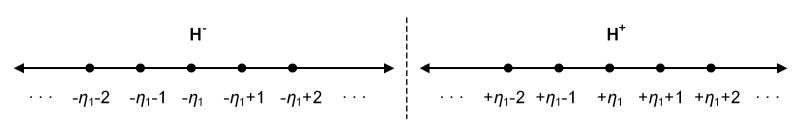Combining the two sets R and H gives us an extended number line with zero in the center. In other words, we can fit the real number line between the halves of the suprareal line, capturing the essence of the ordering relationship between the negative suprareals, the reals, and the positive suprareals.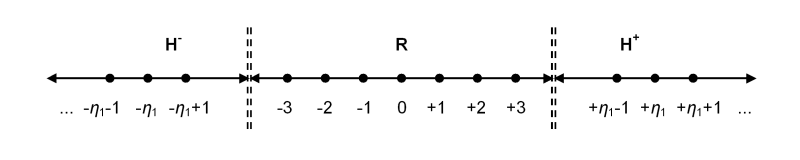As useful as this diagram is, it's important to realize that visualizing the union of the reals and the suprareals (R ∪ H) as a single contiguous extended real number line is misleading, because there is no continuity or "connecting points" between the negative reals and the negative suprareals, and likewise none between the positive reals and the positive suprareals. No matter how far to the right we proceed along the positive reals, we never reach a "greatest positive" real that is adjacent to a "least positive" suprareal. There is no point where the reals "meet" the suprareals, on either the positive or the negative half of the lines. Thus the union of the two sets contains two "gaps", or discontinuities, between them.

## Multiplicative Inverses

Earlier it was implied that defining multiplicative inverses (a reciprocal or division operator) over the suprareals could possibly make them a field. This is not quite correct; what happens is that once multiplicative inverses are introduced into our system, a new kind of number is brought into existence. Given any real x greater than 1, its reciprocal 1/x is a positive real number less than 1 but greater than 0. Similarly, given any positive suprareal h, which by definition is greater than any real, its reciprocal 1/h is a new positive number that is less than any real but still greater than 0. We formalize this with an axiom that defines into existence a specific multiplicative inverse of the suprareals:

While Axiom 8 is correct as shown, the equivalent statement for it is incorrect.

We can define a multiplicative inverse for η1 (i.e., ε1), but this does not imply that every hH has an inverse. For instance, η1+1 does not have an inverse that can be represented as a polynomial over ε1. In fact, it appears that η1 is the only suprareal that has a well-defined inverse.

In order to allow all suprareals to have multiplicative inverses, we would have to allow the inverse suprareals to be polynomials and power series, i.e., series with an infinite number of terms. However, it is not clear at this point what meaning to assign to a sum such as:

s = x1ε11 + x2ε12 + x3ε13 + ...
Specifically, how do we determine whether such a series converges or not?

Until we can answer these questions, we can simply choose the simpler approach for now and restrict the suprareals and i-suprareals to being only polynomials over η1 and ε1.

This means, of course, that suprareals in general do not have multiplicative inverses, and thus the union set HRL does not form a field (so Theorem 19 is false).

Axiom 8.
There exists ε1 = 1/η1, such that 0 < ε1 < x for all xR.
Or, equivalently:
For all hH where h > 0, there exists ε = 1/h,
such that 0 < ε < x for all xR.

This new fundamental constant ε1 and all the other numbers derivable from it are infinitesimal numbers, because they are smaller than any real but still greater than zero. For now, however, we'll simply call them the i-suprareals ("inverse suprareals"). For convenience, we place them into their own set L:

Definition 6.
L = { 1/h : hH }.

Like the suprareals, it appears that every i-suprareal can be represented as a polynomial over powers of ε1:

Theorem FTI.
For all vL,
v = x1ε11 + x2ε12 + x3ε13 + ... + xnε1n,
where x1, x2, ..., xnR, and at least one xj ≠ 0.

As was noted earlier, the suprareals by themselves are not a field (because dividing a suprareal by some other number does not always produce another suprareal), but the union of the suprareals, the reals, and the i-suprareals is indeed a field:

For all u,vHRL, u+vHRL.
Theorem 18b. (Closure over multiplication)
For all u,vHRL, uvHRL.
Theorem 18c. (Closure over multiplicative inversion)
For all u ≠ 0 ∈ HRL, 1/uHRL.
For all uHRL, 0+u = u.
For all uHRL, −u + u = 0.
Theorem 18f. (Multiplicative identity)
For all uHRL, 1•u = u.
Theorem 18g. (Multiplicative inverse)
For all u ≠ 0 ∈ HRL, u1/u = 1.
Theorem 19.
(HRL, +, •) is a field.

To visualize adding the i-suprareals to the extended real number line, note that all of the positive i-suprareals fall between zero and all of the positive reals, and likewise for the negative i-suprareals, which fall between all of the negative reals and zero. So if we visualize the normal real number line as being centered at zero, we can split it into two half-lines and a point, i.e., the negative reals, zero, and the positive reals.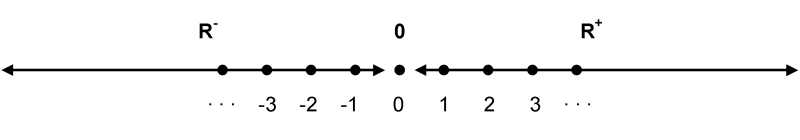The negative i-suprareals are then inserted between the negative half-line and zero, and the positive i-suprareals are inserted between zero and the positive half-line. Positive and negative ε1 are used as the "center points" of the i-suprareal half-lines.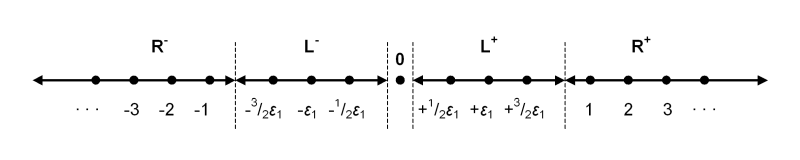We can now show the hierarchy of i-suprareals, reals, and suprareals by listing their half-lines according to the ordering of the points contained within them:

For all positive εL, xR, hH,
−∞ < −h < −x < −ε < 0 < +ε < +x < +h < +∞
H < R < L < 0 < L+ < R+ < H+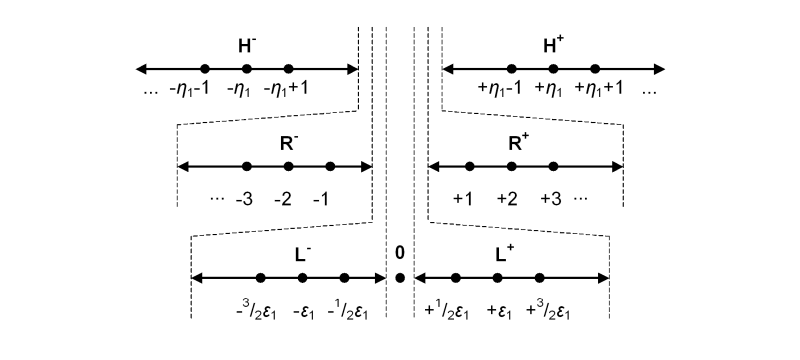## Extending the Numbers

The visualization shown earlier of the reals nested within the suprareals suggests that we can continue to extend the suprareals even further, adding another layer of numbers surrounding the suprareals. If we call the suprareals as they have been defined earlier as η1-numbers, we can likewise define new η2-numbers into existence. To make things a little clearer, we'll rename set H as H1, since it is composed of all the η1-numbers derived from η1.

Just as we defined a fundamental element η1 which is greater than any real number, likewise we now define another fundamental element η2 which is greater than any η1-number (and which is obviously greater than any real number as well):

Axiom 9.
There exists η2H2 such that g < η2 for all gH1.

All the new suprareals derived from the fundamental constant η2 are contained in the new set H2.

We can continue defining more layers of suprareals indefinitely, defining into existence an entire hierarchy of suprareals:

Axiom 9. (Generalized)
There exists ηi+1Hi+1 such that g < ηi+1 for all gHi.
Each ηi is the fundamental constant for all of the suprareals in Hi.

It appears that each higher level of suprareals can be represented as polynomials over powers of the fundamental ηi for that level:

Theorem G-FTSR.
For all gHi, g = u + u1ηi1 + u2ηi2 + u3ηi3 + ... + unηin,
where u, u1, u2, ..., unHi−1, and at least one uj ≠ 0.

All of the Hj sets together form a nested hierarchy of suprareals, where the positive and negative halves of each set Hj encloses set Hj−1, forming a nested grouping all the way down to H1, which encloses R.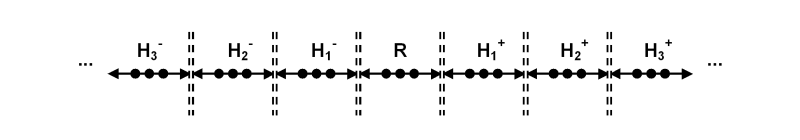Earlier we defined a fundamental i-suprareal constant ε1 corresponding to the fundamental suprareal η1, and likewise we can define an entire hierarchy of sub-infinitesimals εi corresponding to each ηi.

Axiom 10.
There exists εi+1 = 1/ηi+1, such that 0 < εi+1 < εi.
Or, equivalently:
For all giHi+ there exists εi = 1/gi,
such that 0 < εi < εi−1 < x, for all gi−1Hi−1+ for all xR.

All inverse suprareals can be expressed as polynomials over powers of εi:

Theorem G-FTI.
For all gLi, g = v + v1εi1 + v2εi2 + v3εi3 + ... + vnεin,
where v, v1, v2, ..., vnLi−1, and at least one vj ≠ 0.

All of the suprareal sets and i-suprareal sets can be collected together into a single set. This single mega-set, combined with the real numbers, contains all of the reals, all of the suprareals, and all of the i-suprareals:

Definition 7.
RE = RH1L1H2L2 ∪ ...

RE is the set of "extended reals", and contains all of the numbers, real and non-real, defined in our system.

## Nested Hierarchies

Up to this point, we've defined suprareal fundamental constants ηi and their corresponding inverse suprareal constants εi = 1/ηi. But we have not defined an η0. Since the suprareals in Hi form a hierarchy greater than the reals, and the inverse suprareals Li form a hierarchy less than the reals, it seems logical to conclude that we can define H0 to be the set of reals, R. Thus:

Theorem 20. (H0)
H0 = L0 = R.

Since H−0 is simply H0, L0 is just another name for H0, which is just another name for R. Furthermore, since η0 is the fundamental constant of H0, and since its reciprocal 1/η0 is the fundamental contant of L0, and both of these sets are actually the same set R, it follows that η0 is its own inverse in R. That is to say:

Theorem 21.0)
η0 = 1/η0 = 1.

Given all of this, we can simplify our notation somewhat by renaming each set Li as its equivalent set H−i. So where each set Hi has a fundamental contant ηi, likewise each set H−j has a fundamental contant η−j = εj = 1/ηj. This makes the set names Lj redundant, as we now can consider every suprareal, inverse suprareal, or real as a member of some suprareal set Hi.

[This may require some adjustments to the notion that 0 and 1 are not "proper" suprareals, since they are not members of any set Hi where i ≠ 0, but they are members of H0. Taken as a whole, the union of all Hi sets is therefore a field and a "complete" superset of R.]

## Complex Numbers

We can go another step further and extend the suprareals over the complex field. Just as a complex real number is a combination of real and imaginary parts, a complex suprareal is likewise a combination of suprareal and imaginary suprareal parts:

Definition 8.
z = u + iv, for some u,vH1.

Applying this to the entire hierarchy of suprareals, for every suprareal and i-suprareal set Hi there is a corresponding set CHi that extends those suprareals into the complex plane:

Definition 9.
CHi = { z : z = u+iv for all u,vHi } for all iZ.

Note that as a special case CH0 is just C, the standard set of complex numbers.

The final step is to combine all of the complex suprareals, complex i-suprareals, and complex reals into a single set, the extension of the complex numbers:

Definition 10.
CE = CCH1CH−1CH2CH−2 ∪ ...

The diagram below illustrates one way of visualizing set CE. The dashed lines represent the discontinuities between the subsets comprising the extended complex plane. The diagram is simplified, showing only two levels of suprareals and i-suprareals instead of the entire (infinite) hierarchy.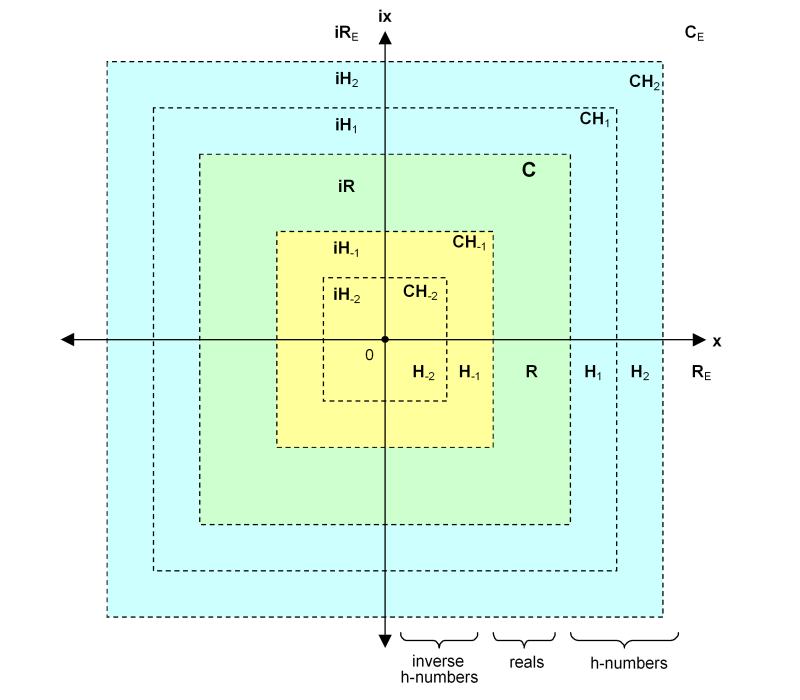## n-Dimensional Numbers

We can also extend the suprareals into quaternion space, creating numbers of the form ih+jg+ku.

Our final step is to extend the suprareals into n-dimensional number spaces. The approach is to define n-tuples <v1v2v3, ...>, where each vi is a member of RE or CE.

## Still Bigger Sets

If we consider that zero is surrounded by the entire set of i-suprareals, the negative i-suprareals on the left and the positive i-suprareals on the right, we could say that the i-suprareals form a "cloud" or "halo" (borrowing a term from non-standard analysis ) of non-real numbers around zero.

Taking this concept one step further, we can combine the i-suprareals with all of the reals in such a way that every real is surrounded by its own halo of i-suprareals:

Definition 11.
RL = R ∪ { x+ε : xR and εL }.

Or, equivalently:

RL = { x+ε : xR and ε ∈ {0} ∪ L }.

This defines set RL having all the reals as members, and for each real x, all of the i-suprareals x+ε surrounding x as well.

We can likewise define a set of the suprareals that includes the i-suprareal halos around each suprareal:

Definition 12.
HL = H ∪ { h+ε : hH and εL }.

Since the i-suprareals are defined in terms of the fundamental constant ε1, which in turn is defined in terms of the fundamental constant η1, we can rewrite the i-suprareals as polynomials over negative powers of η1 (where ε1n1/η1n = η1−n):

For all vL,
v = x1η1−1 + x2η1−2 + ... + xnη1−n,
where x1, x2, ..., xnR, and at least one xj ≠ 0.

Furthermore, the members of HL are sums of suprareals and i-suprareals. It is therefore apparent that the members of this new set can be described as polynomials over all of the integral powers of η1 (positive and negative powers included):

Theorem FTSR-L.
For all hHL,
h = ymη1−m + ... + y2η1−2 + y1η1−1 + x + x1η11 + x2η12 + ... + xnη1n,
where ym, ..., y2, y1, x, x1, x2, ..., xnR, and at least one xj ≠ 0.

And finally, we can combine all of these numbers with the infinitesimals into a single set:

Definition 13.
RU = LRLHL.

Every element in this set is either a real or a suprareals, an i-suprareal, a real plus an i-suprareal, or a suprareal plus an i-suprareal.

## An Uncountable Hierarchy

Up to this point, we have defined a countable hierarchy of suprareals and inverse suprareals, i.e., the sets Hn for every integer n. (Remember that H0 is a special case and is the same as R.)

But we can create an uncountable hierarchy of suprareal sets by defining an suprareal constant for every real:

Axiom 9-U. (Generalized uncountable)
For all xR and x > 0,
there exists ηxHx such that r < ηx for all rR,
and ηx < ηy for all x,yR where x < y.
Axiom 10-U. (Generalized uncountable)
For all ηxHx,
there exists 1/ηx = εxHx such that 0 < εx < r for all rR.

In other words, for every real x there is a suprareal ηx, which is the fundamental constant for an entire set of suprareals Hx. Every suprareal h in Hx can be written as a polynomial over integer powers of ηx, thus h = f(ηx). Furthermore, for every suprareal hx in Hx and every suprareal hy in Hy, hx < hy when x < y.

For example, we can compare various suprareals:

h = fh1) = x0 + x1η11 + x2η12 + ... + xkη1k
g = fg0.5) = y0 + y1η0.51 + y2η0.52 + ... + ymη0.5m
u = fuπ) = z0 + z1ηπ1 + z2ηπ2 + ... + znηπn

Since 0.5 < 1 < π, we know that η0.5 < η1 < ηπ, which tells us that fg0.5) < fh1) < fuπ), and therefore g < h < u.

We can combine all of the Hx sets into a single set:

Definition 14.
HR = { h : hHx } for all xR.

Or, equivalently:

HR = ∪ Hx for all xR.

Since |R| = c, and since |Hx| = c for every for each real x in R, there are c2 suprareals in the union of all the Hx sets. By cardinal exponentiation, c2 = c, so |∪Hx| = c. In other words, HR is the same size as R and H.

## Loose Ends

The definition of the suprareals could be simpler. In particular, there seem to be too many axioms, which could probably be replaced by a more general rule, something like the transfer principle  of non-standard analysis , which would allow most of the arithmetic operators (order, addition, and multiplication) to be defined over the suprareals with a single rule. In particular, Axiom 6 (additive commutativity) and Axiom 7 (multiplicative commutativity) should be theorems instead of axioms.

There is also the question of how operations more complex than arithmetic addition and multiplication may be defined over the suprareals. Analytic functions require the notion of limit, and it is not yet clear how limh→∞ f(h) is to be defined for h in H.

Are the suprareals the same as the hyperreals  of non-standard analysis? They certainly share some of the same properties, notably their ordering relation to the reals. It could be that a given member of RL, being the sum of a real and an i-suprareal x+ε, is equivalent to a non-standard real r and one of the infinitesimals in its halo, with x being the standard part of r, thus x = st(r) and ε = r − st(r).

In other ways, though, the suprareals do not appear to behave like the hyperreals. Specifically, the infinite set of standard real numbers is not definable within internal set theory  (specifically, all infinite sets of IST contain a non-standard element, and all sets of IST containing only standard elements are always finite), yet R is clearly definable as a proper subset of RE and RL. Also, the hyperreals *R do not form a metric space, but it appears that the suprareal sets H, RE, and L ∪ RL ∪ HL do.

Furthermore, reciprocals of non-standard infinitesimals are called infinite numbers (because they are larger than any reals), whereas the suprareals, while also being reciprocals of infinitesimals, are not considered to be numbers with infinite magnitudes, but simply comprise a different set of numbers similar to, but separate from, the reals. This could be just a difference in nomeclature, though, if it turns out that the suprareals and the hyperreals are equivalent. The hyperreals are also called illimited numbers, which is probably the more "correct" term.

Another issue to discuss in more detail is the fact that each region (or level) of suprareals is unconnected to any other region. For example, each h = xηi is greater than every g = yi (for positive reals x,y). Furthermore, each h in Hi is greater than every g in Hi−1. All of these suprareals exist within different, unconnected regions of suprareal space. The suprareals form a highly unconnected set, in fact.

The author does not posess the mathematical expertise to answer these questions definitively, so perhaps others with a deeper understanding of these things can provide better answers.

## References

 Hyperreal numbers
Wikipedia: http://en.wikipedia.org/wiki/Hyperreal_number,
MathWorld: http://mathworld.wolfram.com/HyperrealNumber.html.

 Nonstandard Analysis
Wikipedia: http://en.wikipedia.org/wiki/Nonstandard_analysis,
MathWorld: http://mathworld.wolfram.com/NonstandardAnalysis.html.

 Nonstandard Analysis
Abraham Robinson, 1974, ISBN 0-691-04490-2.

 Transfer Principle
Wikipedia: http://en.wikipedia.org/wiki/Transfer_principle,
MathWorld: http://mathworld.wolfram.com/TransferPrinciple.html.

 Internal Set Theory
Wikipedia: http://en.wikipedia.org/wiki/Internal_set_theory,
MathWorld: http://mathworld.wolfram.com/SaturatedEnlargement.html.

This document: http://david.tribble.com/text/hnumbers.html.